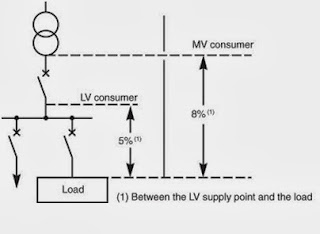## Wednesday, 23 October 2013

### Voltage drop calculation in cablevoltage drop in cable
Cable Voltage Drop
The impedance of circuit conductors is low but not negligible, when carrying load current there is a voltage drop between the origin of the circuit and the load terminals. The correct operation of a load (a motor, lighting circuit, etc.) depends on the voltage at its terminals being maintained at a value close to its rated value. It is necessary therefore to determine the circuit conductors such that at full-load current, the load terminal voltage is maintained within the limits required for correct performance.
This section deals with methods of determining voltage drops, in order to check that:
·         They comply with the particular standards and regulations in force
·         They can be tolerated by the load
·         They satisfy the essential operational requirements

Maximum allowable voltage-drop varies from one country to another. Typical values for LV installations are given below in Figure
 Sr. Type of installations Lighting circuits Other uses (heating and power) 1 A low-voltage service connection from a LV public power distribution network 3% 5% 2 Consumers MV/LV substation supplied from a public distribution MV system 6% 8%

These voltage-drop limits refer to normal steady-state operating conditions and do not apply at times of

motor starting, simultaneous switching (by chance) of several loads, etc. When voltage drops exceed the values shown in Figure, larger cables (wires) must be used to correct the condition.
The value of 8%, while permitted, can lead to problems for motor loads; for example:
In general, satisfactory motor performance requires a voltage within ± 5% of its rated nominal value in steady-state operation,
Starting current of a motor can be 5 to 7 times its full-load value (or even higher). If an 8% voltage drop occurs at full-load current, then a drop of 40% or more will occur during start-up. In such conditions the motor will either:
Stall (i.e. remain stationary due to insufficient torque to overcome the load torque) with consequent over-heating and eventual trip-out
Or accelerate very slowly, so that the heavy current loading (with possibly undesirable low-voltage effects on other equipment) will continue beyond the normal start-up period
Finally an 8% voltage drop represents a continuous power loss, which, for continuous loads will be a significant waste of (metered) energy. For these reasons it is recommended that the maximum value of 8% in steady operating conditions should not be reached on circuits which are sensitive to under-voltage problems
Voltage Drop in Single Phase
An approximate check can be made on voltage drop by means of the following formulae-
For DC and single phase AC two wire systems
Voltage drop = (2xIxLxRxt)/1000
Where I = current in ampere
L = length of cable in meter (one conductor only)
R = resistance of one conductor from table
t = temperature correction factor
Typical example:
Voltage Drop in Three Phase Ac Systems
Voltage drop = (1.732xIxLxRxt)/1000
Note: formula is applied when power factor is unity. Power factor to be considered in load and reactance to be considered
Typical example:
Method-1, millivolts per ampere per metre of run (mV/A/m). This method does not give accurate results for unbalance loads.
Each cable rating in the Tables has a corresponding volt drop figure in millivolts per ampere per metre of run (mV/A/m). Strictly this should be mV/(A m), but here we shall follow the pattern adopted by BS 7671: 1992. To calculate the cable volt drop:
1.-take the value from the volt drop table (mV/A/m)
2. - multiply by the actual current in the cable (NOT the current rating)
3. -multiply by the length of run in metres (circuit length)
4.-divide the result by one thousand (to convert millivolts to volts).

A)    Voltage drop mV/A-m for LV multi-core copper cable
Conductor size  single phase/ PVC/XLPE     three phase /PVC/XLPE
1.5                                           31                    27
2.5                                           20                    16
4                                              12                    10.5
6                                              8                      7
10                                            5                      4
16                                            3                      3
25                                            2                      1.5
35                                            1.5                   1.25
50                                            1                      1
70                                            0.7                   0.7
95                                            0.6                   0.5
120                                          0.5                   0.4
150                                          0.4                   0.3
185                                          0.3                   0.27
240                                          0.25                 0.25
300                                          0.25                 0.2
B) Voltage drop mV/A-m for LV multi-core Aluminium cable
Conductor size  single phase/ PVC/XLPE     three phase /PVC/XLPE
16                                            5                      4.5
25                                            3                      3
35                                            2.5                   2
50                                            1.7                   1.5
70                                            1.2                   1
95                                            1                      0.75
120                                          0.7                   0.6
150                                          0.6                   0.5
185                                          0.5                   0.4
240                                          0.4                   0.3
300                                          0.3                   0.27

Note:
·         There is very less difference between mV/A-m of single core and Multicore.
·         Voltage drop in XLPE cable is higher around 4-5% than PVC
·         Size of the cable is not maximum, it is indicative only.
·         Readers are requested to check with manufactures’ catalogue.
For example-1, if a 4 mm² PVC Sheathed copper cable feeds a 6 kW AC load on 240V and has a length of run of 16 m, we can find the volt drop thus:
The volt drop figure for 4 mm² two-core cable is 12 mV/A/m (from table).
Load current = 6 x 1000/240 = 25A
Total Volt Drop = (mV/A-m drop value x load current x Cable Length)/1000
Voltage drop = (12 x 25 x 16)/1000 = 4.8V
Since the permissible volt drop is 3% of 240 V, which is 12.0 V, the cable in question meets volt drop requirements.

For example-2, if a 1.5 mm² PVC Sheathed copper cable feeds 12 Nos. 3x 36W  luminaries  load on 240V and has a length of run of 35 m, we can find the volt drop thus:
The volt drop figure for 4 mm² two-core cable is 31 mV/A/m (from table).
Load current = 12 x 3 x 36 /240 = 5.4A
Total Volt Drop = (mV/A-m drop value x load current x Cable Length)/1000
Voltage drop = (31 x 5.4 x 35)/1000 = 5.86V

Book reference :
A practical guide to cable installation and tool box talk
Available with book shop and -

Price: Rs. 375/- excluding delivery charges